# Write the truth table for the circuits given in figure

Write the truth table for the circuits given in figure consisting of N(5R gates identify the logic operations (OR, AND, NOT) performed by the two circuits.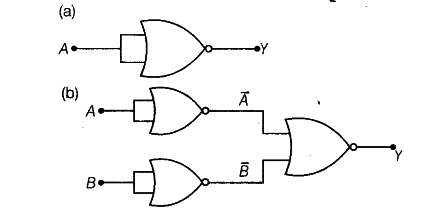(a) Split the gate,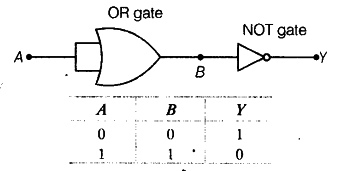B is the output of OR gate and input of NOT gate.
So, the gate resembles to NOT gate as A is input and Y is output.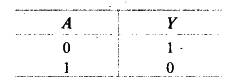(b) Split the gate,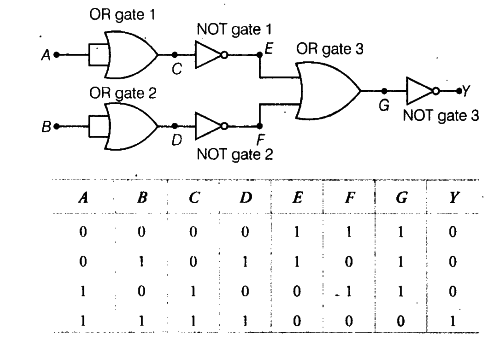C is the output of OR gate 1 and input of NOT gate 1
D is the output of OR gate 2 and input of NOT gate 2
E is the output of NOT gate 1; F is the output of NOT gate 2
G is the output of OR gate 3 and input of the NOT gate 3
The truth table resembles to AND gate as A and B inputs and Y is output.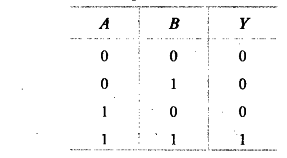This operation is AND gate.